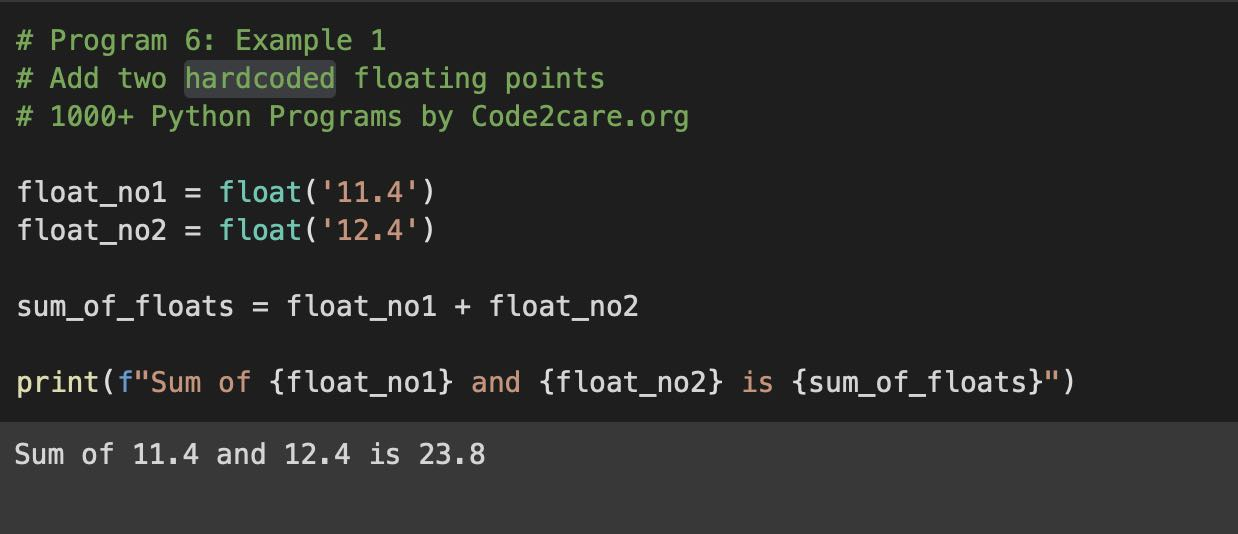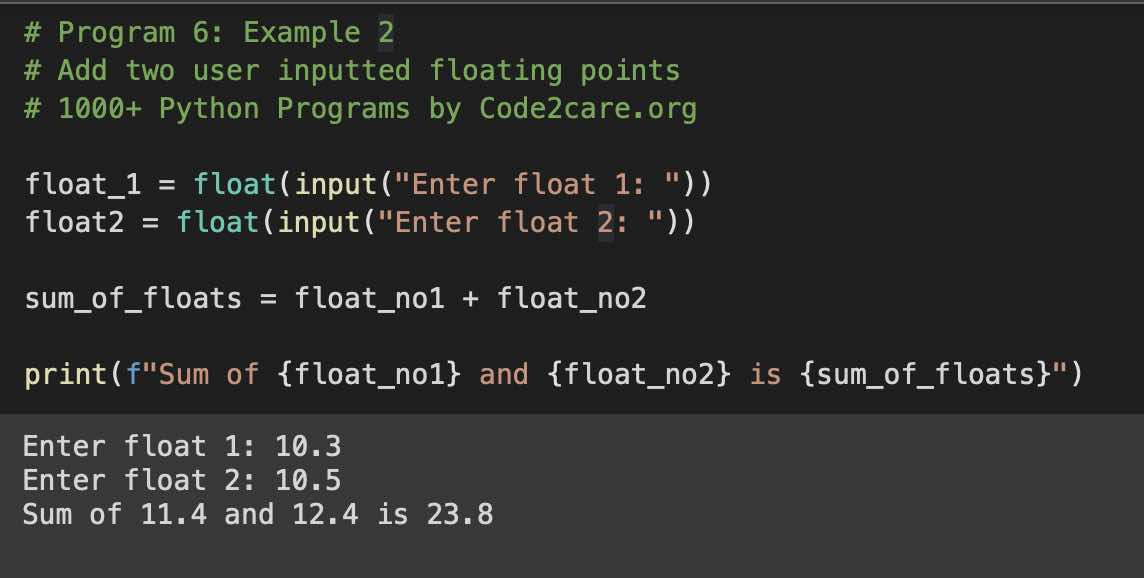# Program 6: Find Sum of Two Floating Numbers - 1000+ Python Programs

Question 6) Write a program to find the sum of two floating numbers in Python.

## Example 1: Hardcoded Floating Points

``````# Program 6: Example 1
# Add two hardcoded floating points
# 1000+ Python Programs by Code2care.org

float_no1 = float('11.4')
float_no2 = float('12.4')

sum_of_floats = float_no1 + float_no2

print(f"Sum of {float_no1} and {float_no2} is {sum_of_floats}")``````
Output:

Sum of 11.4 and 12.4 is 23.8

Explanation:
1. Create a variable float_no1 and store a float value for number 1 using the float function
2. Create a variable float_no2 and store a float value for number 2 using the float function
3. Now, create a third variable where we add these two floats.
4. Print the result using a print function with f-strings.## Example 2: Using user inputs

``````# Program 6: Example 2
# Add two user inputted floating points
# 1000+ Python Programs by Code2care.org

float_1 = float(input("Enter float 1: "))
float2 = float(input("Enter float 2: "))

sum_of_floats = float_no1 + float_no2

print(f"Sum of {float_no1} and {float_no2} is {sum_of_floats}")``````
Output:

Enter float 1: 10.3
Enter float 2: 10.5
Sum of 11.4 and 12.4 is 23.8## Functions used:

1. print(): Built-in function to print a string on the console with f-strings formatting.
2. float(): Built-in function to convert String argument to float value.
3. input(): Built-in function to accept user input